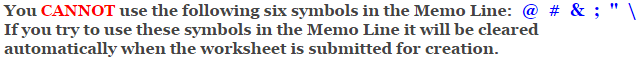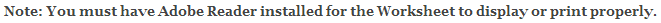# Algebra 2 - Exponential and Logarithmic Functions Worksheets

## Exponential Equations Requiring Logarithms

This Algebra 2 Exponential Equations Requiring Logarithms worksheet will give you exponential equations to solve. You can choose the number of problems you want and the form of the problems.

### Language for the Exponential and Logarithmic Worksheet

 English German Albanian Spanish Swedish Italian French Turkish Polish Norwegian

### Memo Line for the Exponential and Logarithmic Worksheet

You may enter a message or special instruction that will appear on the bottom left corner of the Exponential and Logarithmic Worksheet.### Exponential and Logarithmic Worksheet Answer Page

Now you are ready to create your Exponential and Logarithmic Worksheet by pressing the Create Button.

 Recommended Videos

If You Experience Display Problems with Your Math Worksheet